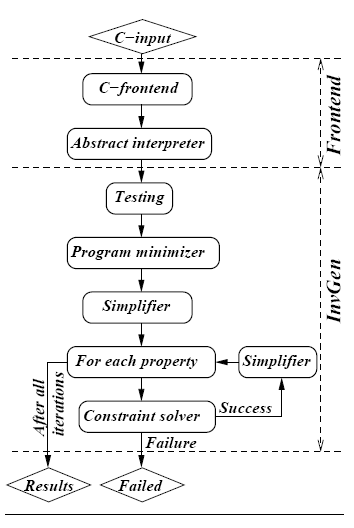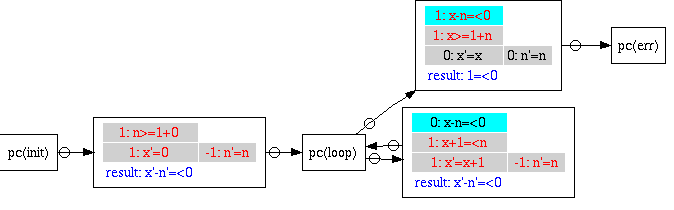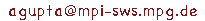##InvGen: An efficient invariant generator

By Ashutosh Gupta and Andrey Rybalchenko

InvGen is an automatic linear arithmetic invariant generator for imperative programs.[PDF]

### Arguments

invgen [option]* file

Option List:
 -templ number Number of conjunct in templates, (disjunctive templates have to be declared in input file) -depth number Depth of execution in testing -timeout number Timeout in seconds -nostrength Disable support of abstract interpreter -dotty Output proof structure in dotty format in input_file.dot -dump_inv Output invariants in input_file.inv -process Runs in process mode -stop-on-bad-input If running in process mode then halts on bad input -help Prints available options

### Input Syntex

InvGen accepts program as a set of transition relations. Example Input files

### Frontend

We also offer a tool Frontend which can translate limited C-language into invgen input language. Its binary also includes abstract interpreter, InterProc.

### Example use

Lets take following example simple.c.
```void simple(int n){
int  x=0;
assume(n>0);
while(x<n){
x++;
}
assert(x<=n);
}```

InvGen input which corresponds to above program is in simple.pl. This file is hand generated for simplicity but the frontend can automatically translate above program into InvGen input format using following command.

```        ./frontend -main simple -o simple.pl -domain 2 simple.c
```

In simple.pl, the start location has given name 'init', the loop head has given name 'loop' and the error location has given name 'err' (click and look inside simple.pl ). InvGen can be used with following command:

```        ./invgen -dotty simple.pl
```
Following is the output of the command
```INVGEN 0.1
creating straight line code between cutpoints...done.

path(): pc(loop) pc(err) {x>=1+n,x'=x,n'=n}
[x>=1+n] [x'=x,n'=n]
path(): pc(loop) pc(loop) {x+1=< n,x'=x+1,n'=n}
[x+1=< n] [x'=x+1,n'=n]
path(): pc(init) pc(loop) {n>=1,x'=0,n'=n}
[n>=1] [x'=0,n'=n]

#Printing Strengthening ==
#pc(loop): [x>=0,n>=1]
#=========================
#Start Tracing for depth 0...#.. done in 0 ms
#=========================
#solving for path to error: path()..# cleared.
#=========================
#contributed facts:
#pc(loop): [x-n=<0]
#=========================
#=========================
#Invariant:
#pc(loop): [x-n=<0]
#Total Solving time: 20 ms
```
Above output shows that InvGen has found an invariant at 'loop' such that error is not reachable. The option '-dotty' makes InvGen produce the proof in dot format, which can be displayed using dotty tool. Following is the proof of correctness of simple.pl found in simple.pl.dot:Facts in cyan background are the invariants. Formulas in gray background are the transition relation of the edge. The formulas in blue color are the proved facts(result) on the destination location. Formulas in red color are ones which are used to prove the result. Note that for 'err' location proved fact is '1=<0' which implicitly means false. Therefore, program is correct.

### Bug Reports

Please email bug reports at: